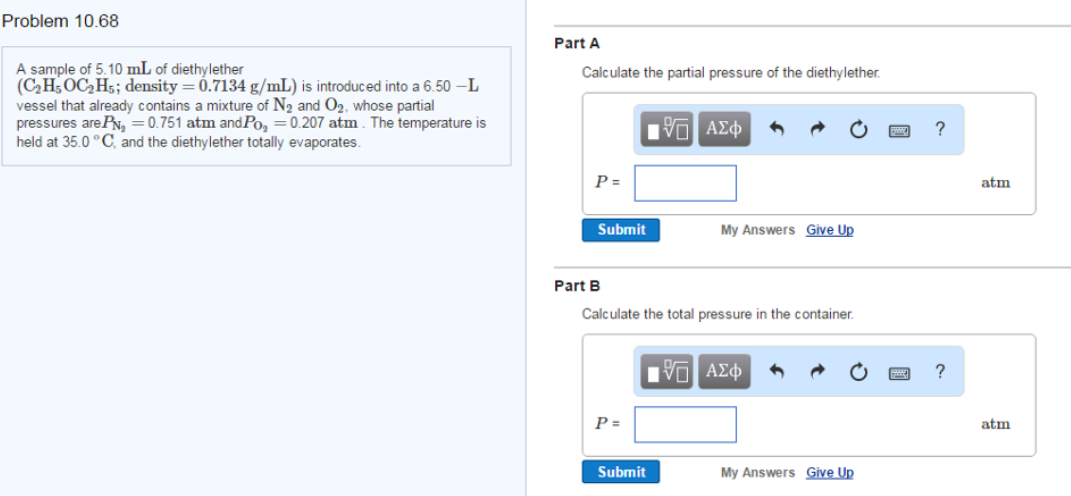# Problem: A sample of 5.10 mL of diethylether (C2H5OC2H5; density = 0.7134 g/mL) is introduced into a 6.50 -L vessel that already contains a mixture of N2 and O2, whose partial pressures are PN2 = 0.751 atm and PO2 = 0.207 atm. The temperature is held at 35.0 °C, and the diethylether totally evaporates. Part A Calculate the partial pressure of the diethylether. Part B Calculate the total pressure in the container.

###### FREE Expert Solution
82% (200 ratings)###### Problem Details

A sample of 5.10 mL of diethylether (C2H5OC2H5; density = 0.7134 g/mL) is introduced into a 6.50 -L vessel that already contains a mixture of N2 and O2, whose partial pressures are PN2 = 0.751 atm and PO2 = 0.207 atm. The temperature is held at 35.0 °C, and the diethylether totally evaporates. Part A Calculate the partial pressure of the diethylether. Part B Calculate the total pressure in the container.